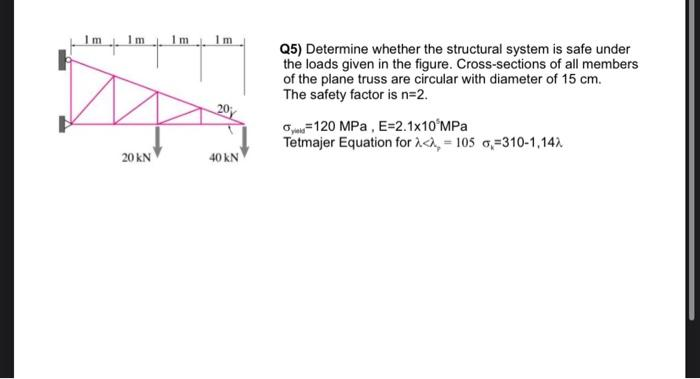# (Solved): Q5) Determine whether the structural system is safe under the loads given in the figure. Cross-sect ...Q5) Determine whether the structural system is safe under the loads given in the figure. Cross-sections of all members of the plane truss are circular with diameter of $$15 \mathrm{~cm}$$. The safety factor is $$n=2$$. $\sigma_{\text {yus }}=120 \mathrm{MPa}, \mathrm{E}=2.1 \times 10^{5} \mathrm{MPa}$ Tetmajer Equation for $$\lambda<\lambda_{p}=105 \quad \sigma_{k}=310-1,14 \lambda$$

We have an Answer from Expert